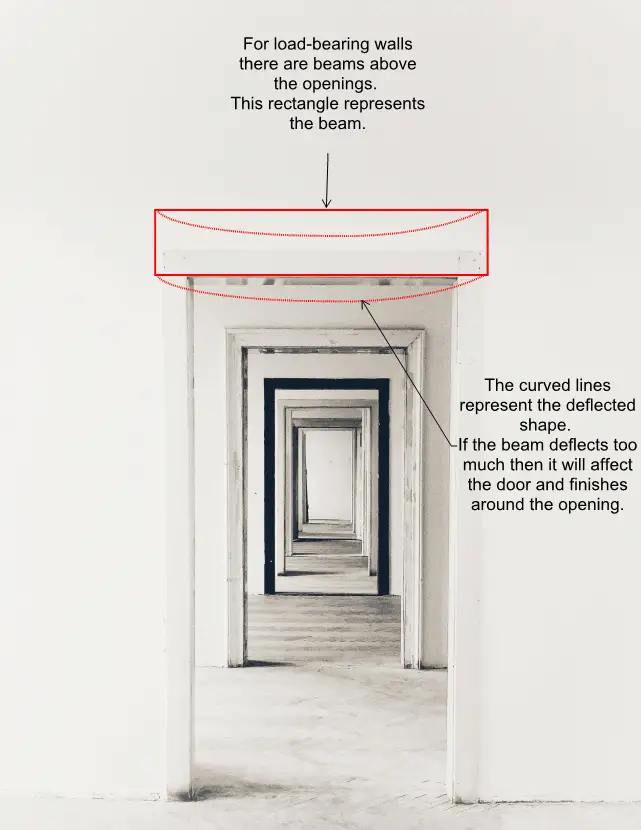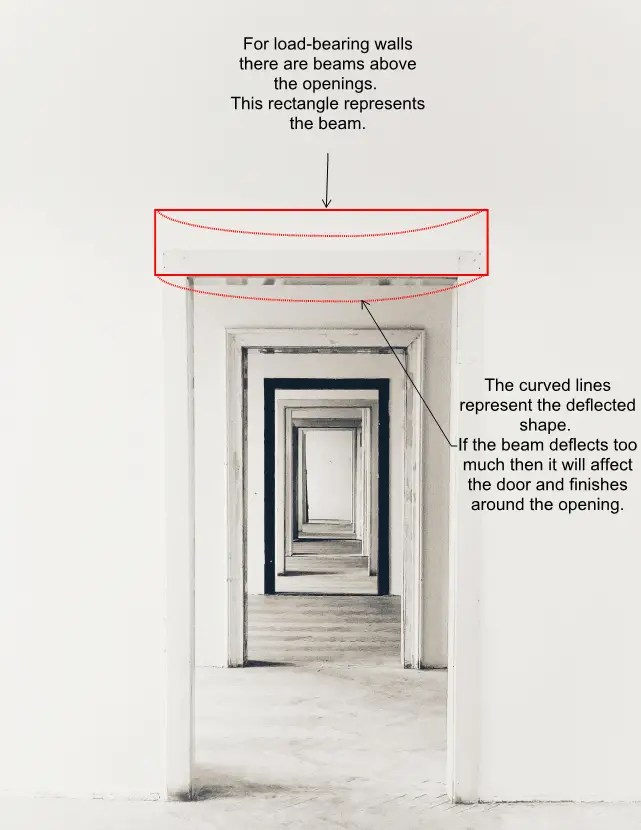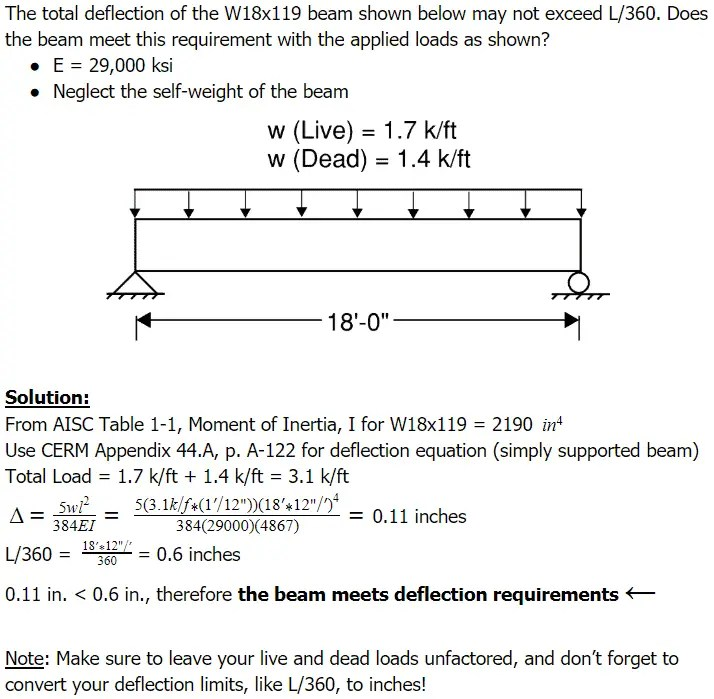Beam Deflection ExamplesBeam Deflection examples and practice problems are important to review when studying for the Civil PE Exam. You will see these problems in the morning session of the test, and on the afternoon session if you are taking the structural depth PE exam. Here is just a brief overview of what you may need to know about this topic for the test.

Definition: Beam Deflection is the amount of downward movement that a beam experiences when loaded, the calculations for which are in the Civil Engineering Reference Manual (CERM) and AISC Steel Construction Manual.

In-Field Beam Deflection Example:Beams are horizontal members that carry vertical loads. When loaded, beams will deflect, or bend downwards vertically. If you have a lot of snow on a roof, for example, then a beam supporting the roof bends downwards because of the load. Deflection calculations determine the amount of that downward movement. Engineering codes limit the amount of deflection allowed for specific applications to values such as L/360, where L is length.

This is an important topic to know because if a beam bends down too much then it will affect the elements below it. We refer to this in civil engineering as serviceability. For example, a beam that is above a bifold door may bend down enough that it prevents a person from opening the door. If the deflection is too large then it may even break the door or other elements.

The calculations for it are reasonably straightforward, and we’ll see that in the examples below. Different loading scenarios require different equations, all of which you can find in the CERM and AISC Steel Manual.

Deflection calculations give values in inches to determine the amount of downward movement. We need to calculate both live load deflection and total load deflection to determine if a beam meets code requirements. Live load deflection is how much a beam will deflect under a full live load alone. Total load deflection includes both live and dead loads, neither of which are factored.

Here are 2 beam deflection examples we’ll review to help you prepare for the PE exam:

• Determine whether a beam meets deflection requirements.Does a beam meet deflection requirements?Review

The calculations for beam deflection are found in the CERM and AISC.

Codes give limits on values for it, which you will be given in the exam.

Input actual numbers into the equations to solve variable change questions.

Similar Study Topics

Mechanics of Materials

Column Buckling

Moment of Inertia

Test Prep Tips

How to Carry Books Into the PE Exam

How Much Does the PE Exam Cost?